# Math in Focus Grade 6 Chapter 3 Lesson 3.3 Answer Key Dividing Decimals

Go through the Math in Focus Grade 6 Workbook Answer Key Chapter 3 Lesson 3.3 Dividing Decimals to finish your assignments.

## Math in Focus Grade 6 Course 1 A Chapter 3 Lesson 3.3 Answer Key Dividing Decimals

### Math in Focus Grade 6 Chapter 3 Lesson 3.3 Guided Practice Answer Key

Complete.

Question 1.
Find 1 ÷ 0.5
Method 1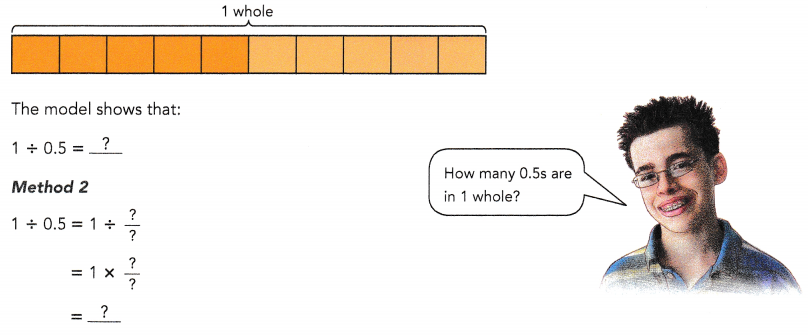There are two 0.5s in 1 whole.

Explanation:
There are two 0.5s are in 1 whole.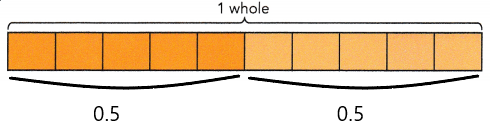Method 2
1 ÷ 0.5 = 1 ÷ $$\frac{5}{10}$$
= 1 × $$\frac{10}{5}$$
= 2.

Question 2.
Find 48 ÷ 0.3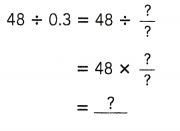48 ÷ 0.3 = 160.

Explanation:
48 ÷ 0.3 = 48 ÷ $$\frac{3}{10}$$
= 48 × $$\frac{10}{3}$$
= 16 × 10
= 160.

Complete.

Question 3.
Complete the model to show the division expression 98 ÷ 0.14.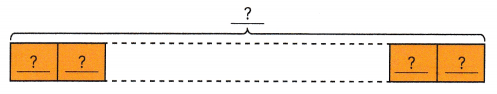98 ÷ 0.14 = 700.

Explanation: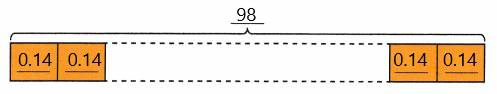Method 2
98 ÷ 0.14 = 98 ÷ $$\frac{14}{100}$$
= 98 × $$\frac{100}{14}$$
= 7 × 100
= 700.

Divide.

Question 4.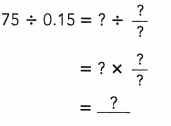75 ÷ 0.15 = 500.

Explanation:
75 ÷ 0.15 = 75 ÷ $$\frac{15}{100}$$
= 75 × $$\frac{100}{15}$$
= 5 × 100
= 500.

Question 5.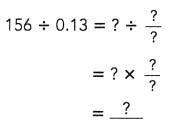156 ÷ 0.13 = 1200.

Explanation:
Given the division expression is 156 ÷ 0.13,
156 ÷ 0.13 = 156 ÷ $$\frac{13}{100}$$
= 156 × $$\frac{100}{13}$$
= 12 × 100
= 1200.

Complete.

Question 6.
Find 0.9 ÷ 0.3.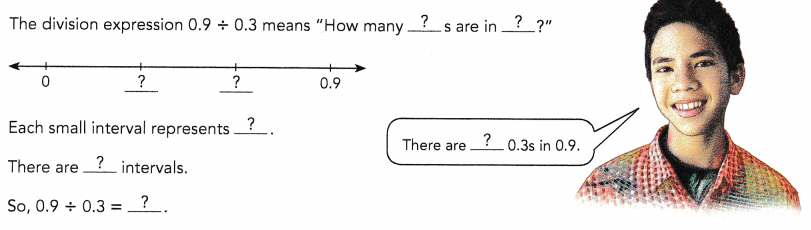There are three 0.3s in 0.9.

Explanation:
Given the division expression is 0.9 ÷ 0.3,
0.9 ÷ 0.3 = $$\frac{9}{10}$$ ÷ $$\frac{3}{10}$$
= $$\frac{9}{10}$$ × $$\frac{10}{3}$$
= 3.
So each small interval represents 0.3 and there are 3 intervals.

Complete.

Question 7.
Find 0.72 ÷ 0.03.
Method 1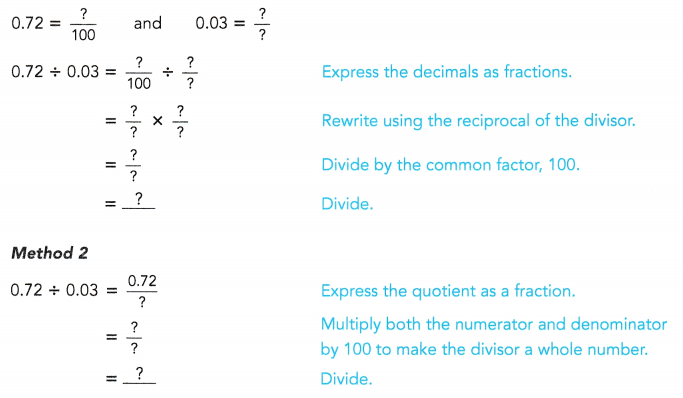0.72 ÷ 0.03 = 24.

Explanation:
Given the division expression is 0.72 ÷ 0.03,
Method 1
0.72 = $$\frac{72}{100}$$  and 0.03 = $$\frac{3}{100}$$
= $$\frac{72}{100}$$ ÷ $$\frac{3}{100}$$
= $$\frac{72}{100}$$ × $$\frac{100}{3}$$
= 24.

Method 2
0.72 ÷ 0.03 = $$\frac{0.72}{0.03}$$
= $$\frac{0.72}{0.03}$$ × $$\frac{100}{100}$$
= $$\frac{72}{3}$$
= 24.

Complete.

Question 8.
Find 0.78 ÷ 0.6.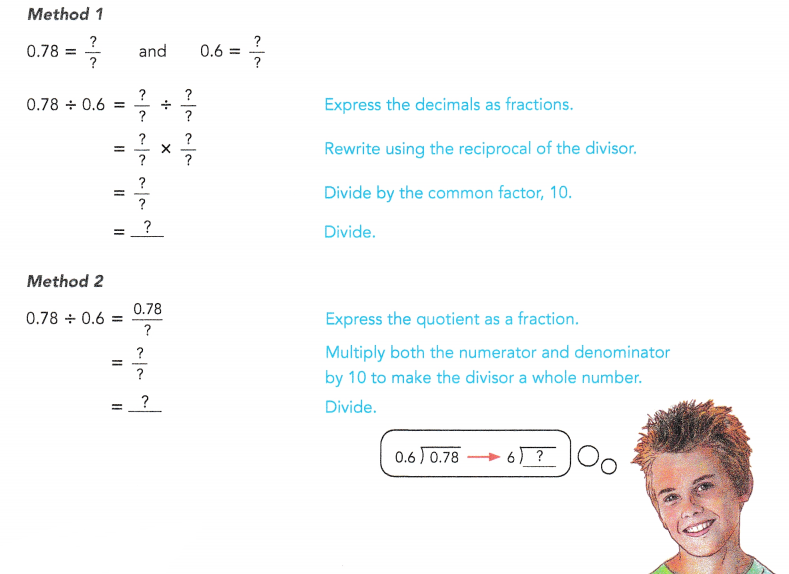0.78 ÷ 0.6 = 1.3.

Explanation:
Given the division expression is 0.78 ÷ 0.6,
Method 1
0.78 = $$\frac{78}{100}$$  and 0.6 = $$\frac{6}{10}$$
= $$\frac{78}{100}$$ ÷ $$\frac{6}{10}$$
= $$\frac{78}{100}$$ × $$\frac{10}{6}$$
= $$\frac{13}{10}$$
= 1.3.

Method 2
0.78 ÷ 0.6 = $$\frac{0.78}{0.6}$$
= $$\frac{0.78}{0.6}$$ × $$\frac{10}{10}$$
= $$\frac{7.8}{6}$$
= 1.3.

Question 9.
Find 6.75 ÷ 0.3.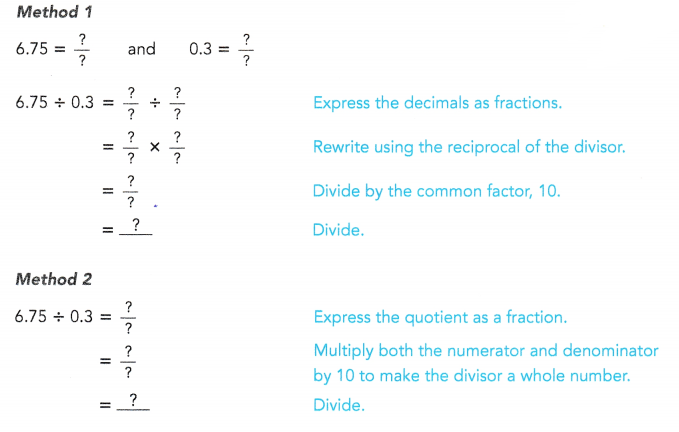Explanation:
Given the division expression is 6.75 ÷ 0.3,
Method 1
6.75 = $$\frac{675}{100}$$  and 0.3 = $$\frac{3}{10}$$
= $$\frac{675}{100}$$ ÷ $$\frac{3}{10}$$
= $$\frac{675}{100}$$ × $$\frac{10}{3}$$
= $$\frac{225}{10}$$
= 22.5.

Method 2
6.75 ÷ 0.3 = $$\frac{6.75}{0.3}$$
= $$\frac{6.75}{0.3}$$ × $$\frac{10}{10}$$
= $$\frac{67.5}{3}$$
= 22.5

### Math in Focus Course 1A Practice 3.3 Answer Key

Write a division expression that represents each model.

Question 1.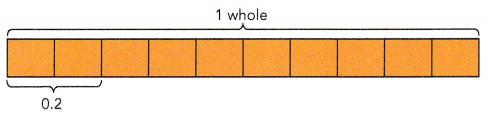1 ÷ 0.2 = 5.

Explanation:
In 1 whole there are five 0.2s, so the division expression will be 1 ÷ 0.2 which is 5.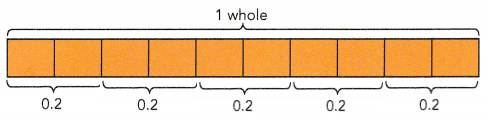Question 2.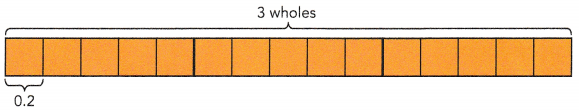3 ÷ 0.2 = 15.

Explanation:
In 3 wholes there are 15 0.2s, so the division expression will be 3 ÷ 0.2 which is 15.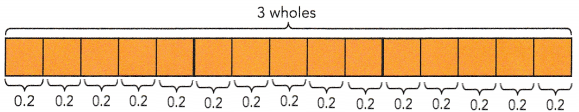Divide.

Question 3.
2 ÷ 0.4
2 ÷ 0.4 = 5.

Explanation:
Given the division expression is 2 ÷ 0.4,
2 ÷ 0.4 = 2 ÷ $$\frac{4}{10}$$
= 2 × $$\frac{10}{4}$$
= 5.

Question 4.
4 ÷ 0.5
4 ÷ 0.5 = 8.

Explanation:
Given the division expression is 4 ÷ 0.5,
4 ÷ 0.5 = 4 ÷ $$\frac{5}{10}$$
= 4 × $$\frac{10}{5}$$
= 8.

Question 5.
5 ÷ 0.2
5 ÷ 0.2 = 25.

Explanation:
Given the division expression is 5 ÷ 0.2,
5 ÷ 0.2 = 5 ÷ $$\frac{2}{10}$$
= 5 × $$\frac{10}{2}$$
= 5 × 5
= 25.

Question 6.
8 ÷ 0.8
8 ÷ 0.8 = 10.

Explanation:
Given the division expression is 8 ÷ 0.8,
8 ÷ 0.8 = 8 ÷ $$\frac{8}{10}$$
= 8 × $$\frac{10}{8}$$
= 10.

Question 7.
9 ÷ 0.3
9 ÷ 0.3 = 30.

Explanation:
Given the division expression is 9 ÷ 0.3,
9 ÷ 0.3 = 9 ÷ $$\frac{3}{10}$$
= 9 × $$\frac{10}{3}$$
= 3 × 10
= 30.

Question 8.
7 ÷ 0.4
7 ÷ 0.4 = 17.5.

Explanation:
Given the division expression is 7 ÷ 0.4,
7 ÷ 0.4 = 7 ÷ $$\frac{4}{10}$$
= 7 × $$\frac{10}{4}$$
= 7 × $$\frac{5}{2}$$
= $$\frac{35}{2}$$
= 17.5.

Question 9.
12 ÷ 0.3
12 ÷ 0.3 = 40.

Explanation:
Given the division expression is 12 ÷ 0.3,
12 ÷ 0.3 = 12 ÷ $$\frac{3}{10}$$
= 12 × $$\frac{10}{3}$$
= 4 × 10
= 40.

Question 10.
42 ÷ 0.7
42 ÷ 0.7 = 60.

Explanation:
Given the division expression is 42 ÷ 0.7,
42 ÷ 0.7 = 42 ÷ $$\frac{7}{10}$$
= 42 × $$\frac{10}{7}$$
= 6 × 10
= 60.

Question 11.
55 ÷ 0.5
55 ÷ 0.5 = 110.

Explanation:
Given the division expression is 55 ÷ 0.5,
55 ÷ 0.5 = 55 ÷ $$\frac{5}{10}$$
= 55 × $$\frac{10}{5}$$
= 11 × 10
= 110.

Question 12.
69 ÷ 0.2
69 ÷ 0.2 = 345

Explanation:
Given the division expression is 69 ÷ 0.2,
69 ÷ 0.2 = 69 ÷ $$\frac{2}{10}$$
= 69 × $$\frac{10}{2}$$
= 69 × 5
= 345.

Question 13.
86 ÷ 0.5
86 ÷ 0.5 = 172

Explanation:
Given the division expression is 86 ÷ 0.5,
86 ÷ 0.5 = 86 ÷ $$\frac{5}{10}$$
= 86 × $$\frac{10}{5}$$
= 86 × 2
= 172.

Question 14.
93 ÷ 0.4

Explanation:
Given the division expression is 93 ÷ 0.4,
93 ÷ 0.4 = 93 ÷ $$\frac{4}{10}$$
= 93 × $$\frac{10}{4}$$
= 93 × $$\frac{5}{2}$$
= $$\frac{465}{2}$$
= 232.5.

Question 15.
1 ÷ 0.02
1 ÷ 0.02 = 50.

Explanation:
Given the division expression is 1 ÷ 0.02,
1 ÷ 0.02 = 1 ÷ $$\frac{2}{100}$$
= 1 × $$\frac{100}{2}$$
= 50.

Question 16.
3 ÷ 0.06
3 ÷ 0.06 = 50.

Explanation:
Given the division expression is 3 ÷ 0.06,
3 ÷ 0.06 = 3 ÷ $$\frac{6}{100}$$
= 3 × $$\frac{100}{6}$$
= $$\frac{100}{2}$$
= 50.

Question 17.
6 ÷ 0.15
6 ÷ 0.15 = 40.

Explanation:
Given the division expression is 6 ÷ 0.15,
6 ÷ 0.15 = 6 ÷ $$\frac{15}{100}$$
= 6 × $$\frac{100}{15}$$
= 2 × $$\frac{100}{5}$$
= 2 × 20
= 40.

Question 18.
7 ÷ 0.35
7 ÷ 0.35 = 20.

Explanation:
Given the division expression is 7 ÷ 0.35,
7 ÷ 0.35 = 7 ÷ $$\frac{35}{100}$$
= 7 × $$\frac{100}{35}$$
= 20.

Question 19.
8 ÷ 0.32
8 ÷ 0.32 = 25.

Explanation:
Given the division expression is 8 ÷ 0.32,
8 ÷ 0.32 = 8 ÷ $$\frac{32}{100}$$
= 8 × $$\frac{100}{32}$$
= 25.

Question 20.
9 ÷ 0.72
9 ÷ 0.72 = 12.5.

Explanation:
Given the division expression is 9 ÷ 0.72,
9 ÷ 0.72 = 9 ÷ $$\frac{72}{100}$$
= 9 × $$\frac{100}{72}$$
= 12.5.

Draw a model to show each division expression.

Question 21.
2 ÷ 0.5
2 ÷ 0.5 = 4.

Explanation:
Given the division expression is 2 ÷ 0.5,
2 ÷ 0.5 = 2 ÷ $$\frac{5}{10}$$
= 2 × $$\frac{10}{5}$$
= 4.

Question 22
5 ÷ o.2
5 ÷ o.2 = 10.

Explanation:
Given the division expression is 5 ÷ o.2,
5 ÷ o.2 = 5 ÷ $$\frac{2}{10}$$
= 5 × $$\frac{10}{5}$$
= 10.

Divide.

Question 23.
36 ÷ 0.36
36 ÷ 0.36 = 100.

Explanation:
Given the division expression is 36 ÷ 0.36,
36 ÷ 0.36 = 36 ÷ $$\frac{36}{100}$$
= 36 × $$\frac{100}{36}$$
= 100.

Question 24.
49 ÷ 0.14
49 ÷ 0.14 = 350.

Explanation:
Given the division expression is 49 ÷ 0.14,
49 ÷ 0.14 = 49 ÷ $$\frac{14}{100}$$
= 49 × $$\frac{100}{14}$$
= 49 × 50
= 350.

Question 25.
56 ÷ 0.28
56 ÷ 0.28 = 200.

Explanation:
Given the division expression is 56 ÷ 0.28,
56 ÷ 0.28 = 56 ÷ $$\frac{28}{100}$$
= 56 × $$\frac{100}{28}$$
= 2 × 100
= 200.

Question 26.
72 ÷ 0.20
72 ÷ 0.20 = 360.

Explanation:
Given the division expression is 72 ÷ 0.20,
72 ÷ 0.20 = 72 ÷ $$\frac{20}{100}$$
= 72 × $$\frac{100}{20}$$
= 72 × 5
= 360.

Question 27.
81 ÷ 0.54
81 ÷ 0.54 = 150.

Explanation:
Given the division expression is 81 ÷ 0.54,
81 ÷ 0.54 = 81 ÷ $$\frac{54}{100}$$
= 81 × $$\frac{100}{54}$$
= 3 × $$\frac{100}{2}$$
= 3 × 50
= 150.

Question 28.
256 ÷ 0.64
256 ÷ 0.64 = 400.

Explanation:
Given the division expression is 256 ÷ 0.64,
256 ÷ 0.64 = 256 ÷ $$\frac{64}{100}$$
= 256 × $$\frac{100}{64}$$
= 4 × 100
= 400.

Question 29.
749 ÷ 0.7
749 ÷ 0.7 = 1,070.

Explanation:
Given the division expression is 749 ÷ 0.7,
749 ÷ 0.7 = 749 ÷ $$\frac{7}{10}$$
= 749 × $$\frac{10}{7}$$
= 107 × 10
= 1,070.

Question 30.
972 ÷ 0.8
972 ÷ 0.8 = 1,215.

Explanation:
Given the division expression is 972 ÷ 0.8,
972 ÷ 0.8 = 972 ÷ $$\frac{8}{10}$$
= 972 × $$\frac{10}{8}$$
= 972 × $$\frac{5}{4}$$
= 243 × 5
= 1,215.

Question 31.
96 ÷ 0.16
96 ÷ 0.16 = 1600.

Explanation:
Given the division expression is 96 ÷ 0.16,
96 ÷ 0.16 = 96 ÷ $$\frac{16}{100}$$
= 96 × $$\frac{100}{16}$$
= 16 × 100
= 1600.

Question 32.
545 ÷ 0.25
545 ÷ 0.25 = 218.

Explanation:
Given the division expression is 545 ÷ 0.25,
545 ÷ 0.25 = 545 ÷ $$\frac{25}{10}$$
= 545 × $$\frac{10}{25}$$
= 545 × $$\frac{2}{5}$$
= 109 × 2
= 218.

Question 33.
0.6 ÷ 0.3
0.6 ÷ 0.3 = 2.

Explanation:
Given the division expression is 0.6 ÷ 0.3,
0.6 ÷ 0.3 = $$\frac{6}{10}$$ ÷ $$\frac{3}{10}$$
= $$\frac{6}{10}$$ × $$\frac{10}{3}$$
= 2.

Question 34.
0.64 ÷ 0.04
0.64 ÷ 0.04 = 64.

Explanation:
Given the division expression is 4 ÷ 0.5,
0.64 ÷ 0.04 = $$\frac{64}{100}$$ ÷ $$\frac{4}{100}$$
= $$\frac{64}{100}$$ × $$\frac{100}{4}$$
= 16.

Question 35.
0.78 ÷ 0.06
0.78 ÷ 0.06 = 13.

Explanation:
Given the division expression is 0.78 ÷ 0.06,
0.78 ÷ 0.06 = $$\frac{78}{100}$$ ÷ $$\frac{6}{100}$$
=$$\frac{78}{100}$$ × $$\frac{100}{6}$$
= 13.

Question 36.
0.85 ÷ 0.5
0.85 ÷ 0.5 = 17.

Explanation:
Given the division expression is 0.85 ÷ 0.5,
0.85 ÷ 0.5 = $$\frac{85}{100}$$ ÷ $$\frac{5}{10}$$
= $$\frac{85}{10}$$× $$\frac{10}{5}$$
= 17.

Question 37.
0.025 ÷ 0.5
0.025 ÷ 0.5 = 0.05.

Explanation:
Given the division expression is 0.025 ÷ 0.5,
0.025 ÷ 0.5 = $$\frac{25}{1000}$$ ÷ $$\frac{5}{10}$$
= $$\frac{25}{1000}$$ × $$\frac{10}{5}$$
= $$\frac{5}{100}$$
= 0.05.

Question 38.
0.816 ÷ 0.34
0.816 ÷ 0.34 = 2.4.

Explanation:
Given the division expression is 0.816 ÷ 0.34,
0.816 ÷ 0.34 = $$\frac{816}{1000}$$ ÷ $$\frac{34}{100}$$
= $$\frac{816}{1000}$$ × $$\frac{100}{34}$$
= $$\frac{24}{10}$$
= 2.4.

Question 39.
4.5 ÷ 0.2
4.5 ÷ 0.2 = 22.5.

Explanation:
Given the division expression is 4.5 ÷ 0.2,
4.5 ÷ 0.2 = $$\frac{45}{10}$$ ÷ $$\frac{2}{10}$$
= $$\frac{45}{10}$$ × $$\frac{10}{2}$$
= $$\frac{45}{2}$$
= 22.5.

Question 40.
8.82 ÷ 0.6
8.82 ÷ 0.6 = $$\frac{882}{100}$$ ÷ $$\frac{6}{10}$$
= $$\frac{882}{100}$$ × $$\frac{10}{6}$$
= $$\frac{147}{10}$$